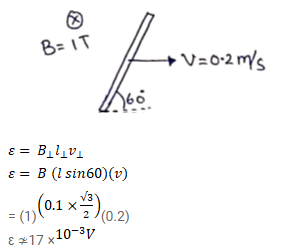# A wire of length 10 cm translates in a direction making and

Question:

A wire of length $10 \mathrm{~cm}$ translates in a direction making and angle of $60^{\circ}$ with its length. The plane of motion is perpendicular to a uniform magnetic field of $1.0 \mathrm{~T}$ that exists in the space. Find the emf induced between the ends of the rod if the speed of translation is $20 \mathrm{~cm} / \mathrm{s}$."

Solution: